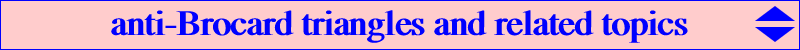The four Brocard triangles AiBiCi are studied here. In this page we define and study the anti-Brocard triangles of the reference triangle ABC (a denomination due to Peter Moses). Definition : a triangle Ti = aibici is said to be an anti-Brocard triangle of ABC when the corresponding Brocard triangle of Ti is ABC itself.T1 = a1b1c1, first anti-Brocard triangle The coordinates of the vertices of T1 are obtained from those of X(385) = a^4 - b^2c^2 : b^4 - c^2a^2 : c^4 - a^2 b^2. It is enough to fix one coordinate and swap the others. This gives : a1 = a^4 - b^2c^2 : c^4 - a^2 b^2 : b^4 - c^2a^2, b1 = c^4 - a^2 b^2 : b^4 - c^2a^2 : a^4 - b^2c^2, c1 = b^4 - c^2a^2 : a^4 - b^2c^2 : c^4 - a^2 b^2, which clearly shows that these points are the G-Hirst inverses of the vertices A1, B1, C1 of the first Brocard triangle. Let f be the affine mapping that transforms ABC into A1B1C1 given by the matrix of the coordinates of A1, B1, C1. f : x :y: z -> a^2 x + c^2 y + b^2 z : c^2 x + b^2 y + a^2 z : b^2 x + a^2 y + c^2 z. By inverting the matrix we find the matrix of the coordinates of a1, b1, c1. This property is not valid if we consider the next three Brocard triangles but remains true for the 5th and 6th Brocard triangles. This will yield the existence of three new related triangles denoted RB2, RB3, RB4 which we call the three Reciprocal Brocard triangles. See below. Obviously, RB1 = T1. Furthermore, the square f^2 of f is the homothety with center the centroid G and ratio (1 - 3 tan2w)/4 where w is the Brocard angle.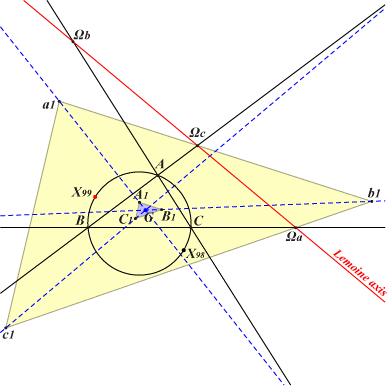Construction of T1 The Lemoine axis (trilinear polar of the Lemoine point K) meets the sidelines of ABC at the centers Ωa, Ωb, Ωc of the Apollonius circles. The parallels at these points to the corresponding sidelines of the first Brocard triangle A1B1C1 bound the requested triangle a1b1c1. The G-Hirst inversion as above gives another construction. Note that X(98), X(99) are the circumcenter and Lemoine point of T1 respectively. A lot of other centers of T1 are listed in ETC. See at X(5939), X(5976). As mentioned there, if X(n) is a center of ABC then its counterpart in a1b1c1is f-1(X(n)). *** There is one and only one isogonal pK with respect to T1 which is a circum-cubic of ABC. It is K699 which is also a pK with respect to ABC. See also K740, an analogous nK0. Properties of T1 • T1 is triply perspective with ABC at X(1916) and its Brocardians, • T1 is orthologic with ABC at X(98) and its counterpart X(5999) in T1, • T1 is parallelogic with ABC at X(99) and X(385), • T1 is indirectly similar with ABC, • T1 is homothetic to A1B1C1 at X(2) and orthologic to A1B1C1 at X(147), X(1352), • T1 is perspective with A2B2C2 at X(2), • T1 is perspective with the cevian triangle of P for any P on the cubic K738 = pK(X76, X3978) passing through X(2), X(6), X(75), X(76), X(290), X(325), X(698), X(1502), X(1916), • T1 is perspective with the anticevian triangle of P for any P on the cubic K739 = pK(X385, X6) passing through X(2), X(6), X(83), X(194), X(239), X(287), X(385), X(732), X(894), X(1916), X(3225).T2 = a2b2c2, second anti-Brocard triangles There are two triangles a2b2c2 whose second Brocard triangle is ABC. They are represented in red and blue on the figure.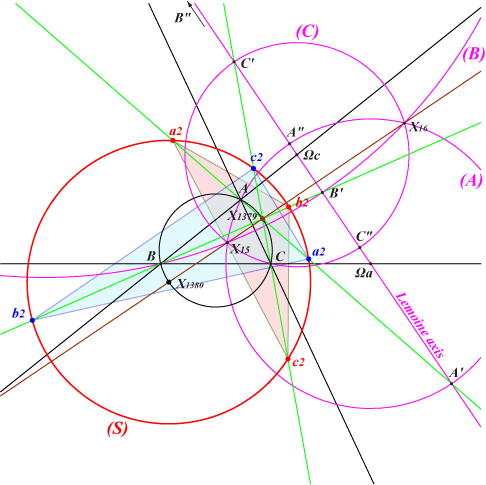Construction of T2 The second Brocard triangle A2B2C2 (of ABC) and ABC share the same Brocard axis which must also be the Brocard axis of T2. Since the Brocard circle of T2 must be the circumcircle (O) of ABC, the Lemoine point of T2 must be K' = X(1379) and its circumcenter must be O' = X(1380). K' is the point on (O) such that K = X(6) is on the segment OK'. (S) is the Schoute circle with center O' hence orthogonal to the Apollonius circles (A), (B), (C). The line AK' meets the Lemoine axis at A' lying on (A) and whose antipode on (A) is A". The points B', C' and B", C" are defined similarly. This same line AK' meets (S) at two points a2. One of them being chosen, the corresponding vertices of T2 are : b2 = BK' /\ a2C" and c2 = CK' /\ a2B". The coordinates of these points are complicated.See the note : To Find the Triangles of Which a Given Triangle is Brocard's Second Triangle, G. Wotherspoon, The Mathematical Gazette, vol. 18, No. 227 (Feb. 1934), pp. 47-48, where a rather complicated solution is given.T3 = a3b3c3, third anti-Brocard triangle (pending)T4 = a4b4c4, fourth anti-Brocard triangle The fourth Brocard triangle A4B4C4 of ABC and ABC share the same Lemoine point K which must be also the Lemoine point of T4. The circumcenter of A4B4C4 is the midpoint X(381) of GH and its circumcircle is the orthocentroidal circle.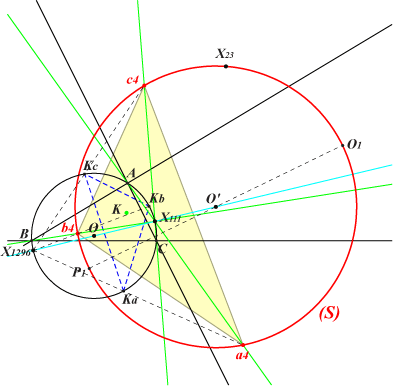Construction of T4 Let KaKbKc be the circumcevian triangle of K. The lines AX(111) and KaX(1296) meet at a4. The other vertices b4, c4 are defined likewise. The coordinates of a4 are : a^2 (a^2+b^2-3 b c+c^2) (a^2+b^2+3 b c+c^2), b^2 (a^2+b^2-2 c^2) (-5 a^2+b^2+c^2), c^2 (a^2-2 b^2+c^2) (-5 a^2+b^2+c^2). Note that X(111) is the centroid of T4, X(1296) is its orthocenter hence the reflection O' of O in X(111) is its circumcenter. Its circumcircle (S) contains X(23) which is actually the Parry point of T4. The Fermat points of T4 are the isodynamic points X(15), X(16) of ABC. K640 is a focal cubic passing through the vertices of T4. It is the Brocard (second) cubic K018 for this triangle.Properties of T4 • T4 is perspective with the antipedal, circumcevian, circumanticevian triangles of X(6) and with the cevian, anticevian, circumanticevian triangles of X(111), • T4 is orthologic with the circumcevian triangle of X(6) at X(1296), O1 (the point X(74) of T4) and also with the antipedal triangle of any point on the circum-conic passing through G and K, • T4 is parallelogic with the circumcevian triangle of X(6) at X(111), P1 (the point X(110) of T4) and also with the antipedal triangle of any point on the circum-conic passing through X(2), X(1296), • T4 is directly similar with A4B4C4, the pedal and circumcevian triangles of X(187), the antipedal triangle of X(671), • T4 is indirectly similar with the pedal and circumcevian triangles of X(6), the antipedal triangle of X(2).RB2, RB3, RB4, RT4 : Reciprocal Brocard triangles The matrices of the vertices of the 2nd, 3rd, 4th Brocard triangles and T4 are inverted as above giving four matrices corresponding to the four Reciprocal Brocard triangles RB2 = u2 : v2 : w2, RB3 = u3 : v3 : w3, RB4 = u4 : v4 : w4 and RT4 = u5 : v5 : w5 with : u2 = -a^4 - b^2 c^2 + b^4 + c^4 : b^2 (a^2 + b^2 - 2 c^2) : c^2 (a^2 - 2 b^2 + c^2), u3 = b^2 c^2 (a^2 - b c) (a^2 + b c) : b^4 (c^2 - a b) (c^2 + a b) : c^4 (b^2 - a c) (b^2 + a c), u4 = -a^4 - b^2 c^2 + b^4 + c^4 : (-a^2 + b^2 + c^2) (a^2 + b^2 - 2 c^2) : (-a^2 + b^2 + c^2) (a^2 - 2 b^2 + c^2), u5 = a^2 - 2 b^2 - 2 c^2 : -5 a^2 + b^2 + c^2 : -5 a^2 + b^2 + c^2, the other coordinates being obtained cyclically. Recall that RB1 is the first anti-Brocard triangle T1. The following table gives some properties of these four triangles in relation with all the Brocard related triangles. Notations : 1P, 3P : simply, triply perspective; H, O, P : homothetic, orthologic, parallelogic, DS, IS : directly, indirectly similar.RB2 RB3 RB4 RT4 ABC 1P, H, O 3P, H, O 1P, P 1P Brocard1 1P, O, P O, P, IS P Brocard2 1P Brocard3 1P Brocard4 1P, O, P, IS 1P T1=RB1 3P, O, P, IS O, P, IS P RB2 1P, H, O P RB3 1P, H, O P RB4 P P P T4 1P O, P, IS 1PT5 = a5b5c5 and T6 = a6b6c6, fifth and sixth anti-Brocard triangles The 5th and 6th anti-Brocard triangles T5 and T6 have matrices the inverses of those of the 5th and 6th Brocard triangles respectively. Their A-vertices are : a5 = (a^2+b^2) (a^2+c^2) : b^4 : c^4 a6 = a^2 (a^2-b c) (a^2+b c) : -a^2 b^4+b^6-a^2 b^2 c^2-b^4 c^2+a^2 c^4+b^2 c^4 : a^2 b^4-a^2 b^2 c^2+b^4 c^2-a^2 c^4-b^2 c^4+c^6.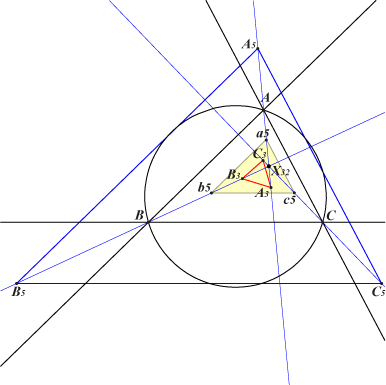T5 is homothetic to ABC and A5B5C5 at X(32) and perspective to A3B3C3 at X(32) as well. More precisely, T5 is the image of ABC under the homothety with center X(32), ratio sin(w) / sin(3w). T5 is orthologic with ABC, A1B1C1, A5B5C5, A6B6C6, a1b1c1 and with the cevian triangle of any point on the Lucas cubic K007. T5 is parallelogic hence inversely similar with A1B1C1, A6B6C6 and a1b1c1. T5 is also orthologic (resp. parallelogic) with the circumcevian triangle of any point on K003 (resp. K024). Construction of T5 It follows that a5 can be seen as the intersection of the lines AX(32) and A'X(83) where A' is the midpoint of BC.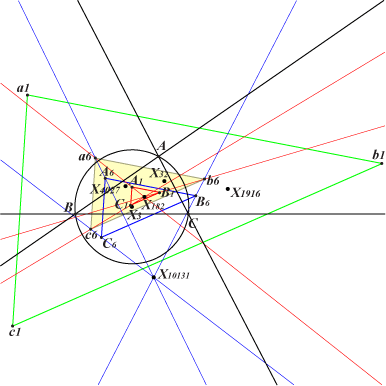T6 is homothetic to A1B1C1 at X(182), to A6B6C6 at X(10131), to a1b1c1 at X(4027). T6 is orthologic with ABC, A1B1C1, A5B5C5, A6B6C6, a1b1c1, a5b5c5 and with the cevian triangle of any point on the cubic pK(X2, X325) which passes through X(2), X(98), X(147), X(325). T6 is parallelogic hence inversely similar with ABC, A5B5C5 and a5b5c5. T6 is also parallelogic with the cevian triangle of any point on pK(X2, X523) which passes through X(2), X(99), X(148), X(523). Construction of T6 It follows that a6 can be seen as the intersection of the parallel at X(1691) and the perpendicular at X(2456) to the sideline BC. Hence the circumcircle of T6 is the circle with diameter X(1691)X(2456) and center X(182).